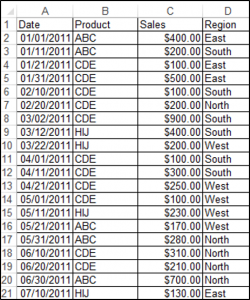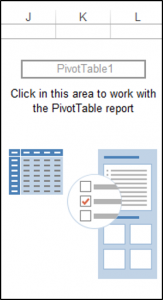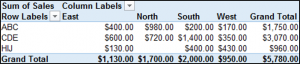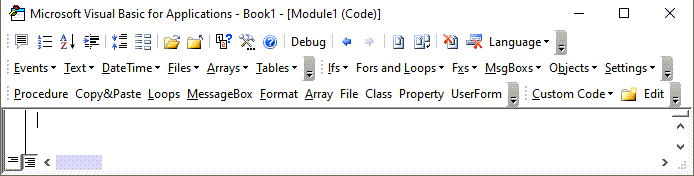# VBA Guide to Pivot Tables

This tutorial will demonstrate how to work with Pivot Tables using VBA.

Pivot Tables are data summarization tools that you can use to draw key insights and summaries from your data. Let’s look at an example: we have a source data set in cells A1:D21 containing the details of products sold, shown below:## Using GetPivotData to Obtain a Value

Assume you have a PivotTable called PivotTable1 with Sales in the Values/Data Field, Product as the Rows field and Region as the Columns field. You can use the PivotTable.GetPivotData method to return values from Pivot Tables.

The following code will return \$1,130.00 (the total sales for the East Region) from the PivotTable:

``````MsgBox ActiveCell.PivotTable.GetPivotData("Sales", "Region", "East")
``````

In this case, Sales is the “DataField”, “Field1” is the Region and “Item1” is East.

The following code will return \$980 (the total sales for Product ABC in the North Region) from the Pivot Table:

``MsgBox ActiveCell.PivotTable.GetPivotData("Sales", "Product", "ABC", "Region", "North")``

In this case, Sales is the “DataField”, “Field1” is Product, “Item1” is ABC, “Field2” is Region and  “Item2” is North.

You can also include more than 2 fields.

The syntax for GetPivotData is:

GetPivotData (DataFieldField1Item1Field2Item2…) where:

Parameter Description
Datafield Data field such as sales, quantity etc. that contains numbers.
Field 1 Name of a column or row field in the table.
Item 1 Name of an item in Field 1 (Optional).
Field 2 Name of a column or row field in the table (Optional).
Item 2 Name of an item in Field 2 (Optional).

## Creating a Pivot Table on a Sheet

In order to create a Pivot Table based on the data range above, on cell J2 on Sheet1 of the Active workbook, we would use the following code:

``````Worksheets("Sheet1").Cells(1, 1).Select

ActiveWorkbook.PivotCaches.Create(SourceType:=xlDatabase, SourceData:= _

"Sheet1!R1C1:R21C4", Version:=xlPivotTableVersion15).CreatePivotTable _

TableDestination:="Sheet1!R2C10", TableName:="PivotTable1", DefaultVersion _

:=xlPivotTableVersion15

Sheets("Sheet1").Select``````

The result is:## Creating a Pivot Table on a New Sheet

In order to create a Pivot Table based on the data range above, on a new sheet, of the active workbook, we would use the following code:

``````Worksheets("Sheet1").Cells(1, 1).Select

ActiveWorkbook.PivotCaches.Create(SourceType:=xlDatabase, SourceData:= _

"Sheet1!R1C1:R21C4", Version:=xlPivotTableVersion15).CreatePivotTable _

TableDestination:="Sheet2!R3C1", TableName:="PivotTable1", DefaultVersion _

:=xlPivotTableVersion15

Sheets("Sheet2").Select``````

## Adding Fields to the Pivot Table

You can add fields to the newly created Pivot Table called PivotTable1 based on the data range above. Note: The sheet containing your Pivot Table, needs to be the Active Sheet.

To add Product to the Rows Field, you would use the following code:

``````ActiveSheet.PivotTables("PivotTable1").PivotFields("Product").Orientation = xlRowField

ActiveSheet.PivotTables("PivotTable1").PivotFields("Product").Position = 1``````

To add Region to the Columns Field, you would use the following code:

``````ActiveSheet.PivotTables("PivotTable1").PivotFields("Region").Orientation = xlColumnField

ActiveSheet.PivotTables("PivotTable1").PivotFields("Region").Position = 1``````

To add Sales to the Values Section with the currency number format, you would use the following code:

``````ActiveSheet.PivotTables("PivotTable1").AddDataField ActiveSheet.PivotTables( _

"PivotTable1").PivotFields("Sales"), "Sum of Sales", xlSum

With ActiveSheet.PivotTables("PivotTable1").PivotFields("Sum of Sales")

.NumberFormat = "\$#,##0.00"

End With``````

The result is:## Changing the Report Layout of the Pivot Table

You can change the Report Layout of your Pivot Table. The following code will change the Report Layout of your Pivot Table to Tabular Form:

``ActiveSheet.PivotTables("PivotTable1").TableStyle2 = "PivotStyleLight18"``

## Deleting a Pivot Table

You can delete a Pivot Table using VBA. The following code will delete the Pivot Table called PivotTable1 on the Active Sheet:

``````ActiveSheet.PivotTables("PivotTable1").PivotSelect "", xlDataAndLabel, True
Selection.ClearContents``````

Stop searching for VBA code online. Learn more about AutoMacro - A VBA Code Builder that allows beginners to code procedures from scratch with minimal coding knowledge and with many time-saving features for all users!## Format all the Pivot Tables in a Workbook

You can format all the Pivot Tables in a Workbook using VBA. The following code uses a loop structure in order to loop through all the sheets of a workbook, and formats all the Pivot Tables in the workbook:

``````Sub FormattingAllThePivotTablesInAWorkbook()
Dim wks As Worksheet
Dim wb As Workbook
Set wb = ActiveWorkbook
Dim pt As PivotTable

For Each wks In wb.Sheets
For Each pt In wks.PivotTables
pt.TableStyle2 = "PivotStyleLight15"
Next pt
Next wks

End Sub``````

## Removing Fields of a Pivot Table

You can remove fields in a Pivot Table using VBA. The following code will remove the Product field in the Rows section from a Pivot Table named PivotTable1 in the Active Sheet:

``````ActiveSheet.PivotTables("PivotTable1").PivotFields("Product").Orientation = _
xlHidden``````

## Creating a Filter

A Pivot Table called PivotTable1 has been created with Product in the Rows section, and Sales in the Values Section. You can also create a Filter for your Pivot Table using VBA. The following code will create a filter based on Region in the Filters section:

``````ActiveSheet.PivotTables("PivotTable1").PivotFields("Region").Orientation = xlPageField

ActiveSheet.PivotTables("PivotTable1").PivotFields("Region").Position = 1``````

To filter your Pivot Table based on a Single Report Item in this case the East region, you would use the following code:

``````ActiveSheet.PivotTables("PivotTable1").PivotFields("Region").ClearAllFilters

ActiveSheet.PivotTables("PivotTable1").PivotFields("Region").CurrentPage = _

"East"``````

Let’s say you wanted to filter your Pivot Table based on multiple regions, in this case East and North, you would use the following code:

``````ActiveSheet.PivotTables("PivotTable1").PivotFields("Region").Orientation = xlPageField

ActiveSheet.PivotTables("PivotTable1").PivotFields("Region").Position = 1

ActiveSheet.PivotTables("PivotTable1").PivotFields("Region"). _
EnableMultiplePageItems = True

With ActiveSheet.PivotTables("PivotTable1").PivotFields("Region")

.PivotItems("South").Visible = False

.PivotItems("West").Visible = False

End With``````

VBA Programming | Code Generator does work for you!

``ActiveSheet.PivotTables("PivotTable1").PivotCache.Refresh``